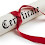### Engineering Mechanics(Civil 1-2)

OBJECTIVE:
This course will serve as a basic course by introducing the concepts of basic mechanics which will help as a foundation to various courses.

UNIT – I
INTRODUCTION OF ENGINEERING MECHANICS – Basic concepts -System of Forces – Moment of Forces and its Application – Couples and Resultant of Force System –Equilibrium of System of Forces - Degrees of Freedom – Free body diagrams –Types of Supports – Support reactions for beams with different types of loading – concentrated, uniformly distributed and uniformly varying loading.

UNIT – II
FRICTION : Types of friction– laws of Friction – Limiting friction- Cone of limiting friction– static and Dynamic Frictions – Motion of bodies – Wedge, Screw jack and differential Screw jack.

UNIT – III
CENTROID AND CENTER OF GRAVITY: Centroids of simple figures – Centroids of Composite figures – Centre of Gravity of bodies – Area moment of Inertia - Parallel axis and perpendicular axis theorems - Moments of Inertia of Composite Figures.
MASS MOMENT OF INERTIA: Moment of Inertia of Simple solids – Moment of Inertia of composite masses.( Simple problems only)

UNIT – IV
KINEMATICS: Rectilinear and Curvi linear motion – Velocity and Acceleration – Motion of A Rigid Body – Types and their Analysis in Planar Motion.
KINETICS : Analysis as a particle and Analysis as a Rigid Body in Translation – Central Forces of motion – Equations of Plane Motion – Fixed Axis Rotation – Rolling Bodies – Work Energy Method – Equation for  Translation – Work Energy application to Particle Motion, Connection System – Fixed axis Rotation and Plane Motion.

UNIT – V
ANALYSIS OF PERFECT FRAMES: Types of frames – cantilever frames and simply supported frames – Analysis of frames using method of joints, method of sections and tension coefficient method for vertical loads, horizontal loads and inclined loads.
MECHANICAL VIBRATIONS: Definitions, Concepts-Simple Harmonic motion-Free vibrations-Simple Compound and Torsional pendulum Numerical problems

Study Material's For Engineering Mechanics

Unit-I:

Unit-II:

Unit-III:

Unit-IV:
1.